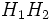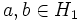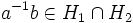# Product formula

This article describes a natural isomorphism between two structures or between a family of structures

## Statement

### Set-theoretic version

Suppose$H_1, H_2 \le G$ are subgroups. Then, there is a natural bijection between the left coset spaces:$|H_1/(H_1 \cap H_2)| \leftrightarrow |H_1H_2/H_2|$.

Note that while$H_1 \cap H_2$, being an intersection of subgroups, is a subgroup,$H_1H_2$ is not necessarily a subgroup.

### Numerical version

Let$H_1$ and$H_2$ be two subgroups of a finite group$G$. Then:$|H_1H_2||H_1 \cap H_2| = |H_1||H_2|$

Here$H_1H_2$ is the product of subgroups.

## Related facts

• Second isomorphism theorem: This is a stronger formulation of the set-theoretic version, which holds when both the groups in the denominator are normal in the respective numerators. In this case, the natural bijection turns out to be an isomorphism.

### Corollaries

• Index of intersection of permuting subgroups divides product of indices: If$HK = KH$, then the index of$H \cap K$ divides the product of the index of$H$ and the index of$K$.
• Index satisfies transfer inequality: This states that if$H, K \le G$, then$[K:H \cap K] \le [G:H]$.
• Index satisfies intersection inequality: This states that if$H, K \le G$ are subgroups, then$[G:H \cap K] \le [G:H][G:K]$.

## Facts used

1. Subgroup containment implies coset containment: If$H \le K \le G$ are subgroups, then every left coset of$H$ is contained in a left coset of$K$.
2. Lagrange's theorem

## Proof

### Proof of the set-theoretic version

Given: A group$G$, and subgroups$H_1, H_2 \le G$.

To prove: There is a natural bijection between the coset spaces$H_1/(H_1 \cap H_2)$ and$H_1H_2/H_2$.

Proof: We first define the map:$\varphi: H_1/(H_1 \cap H_2) \to H_1H_2/H_2$

as follows:$\varphi(g(H_1 \cap H_2)) = gH_2$.

In other words, it sends each coset of$H_1 \cap H_2$ to the coset of$H_2$ containing it.

• The map sends cosets to cosets: Note first that if two elements are in the same coset of$H_1 \cap H_2$, they are in the same coset of$H_2$. Thus, the map sends cosets of$H_1 \cap H_2$ to cosets of$H_2$. (This is fact (1)).
• The map is well-defined with the specified domain and co-domain: Further, if$g \in H_1$, then$gH_2 \subseteq H_1H_2$. In other words, if the original coset is in$H_1$, the new coset is in$H_1H_2$. Thus, the map$\varphi$ is well-defined from$H_1/(H_1 \cap H_2)$ to$H_1H_2/H_2$.
• The map is injective: Finally,$\varphi(a(H_1 \cap H_2)) = \varphi(b(H_1 \cap H_2))$. That means that$aH_2 = bH_2$, forcing$a^{-1}b \in H_2$. But we anyway have$a,b \in H_1$, so$a^{-1}b \in H_1 \cap H_2$, forcing that$a$ and$b$ are in the same coset of$H_1 \cap H_2$. Thus,$a(H_1 \cap H_2) = b(H_1 \cap H_2)$.
• The map is surjective: Any left coset of$H_2$ in$H_1H_2$ can be written as$gH_2$ where$g \in H_1H_2$. Thus, we can write$g = ab$ where$a \in H_1, b \in H_2$. Then,$gH_2 = abH_2 = a(bH_2) = aH_2$, with$a \in H_1$. Thus,$gH_2 = \varphi(a(H_1 \cap H_2))$.

### Proof of the numerical version using the set-theoretic version

The numerical version follows by combining the set-theoretic version with Lagrange's theorem:$|H_1/(H_1 \cap H_2)| = |H_1H_2/H_2|$.

Notice that the left side measures the number of cosets of$H_1 \cap H_2$ in$H_1$. Since all these cosets are disjoint and have size equal to$H_1 \cap H_2$, the left side is$|H_1|/|H_1 \cap H_2|$. Similarly, the right side is$|H_1H_2|/|H_2|$. This yields:$\frac{|H_1|}{|H_1 \cap H_2|} = \frac{|H_1H_2|}{|H_2|}$

which, upon rearrangement, gives the product formula.

(Note: Although for the left side, we can quote Lagrange's theorem to say that$|H_1/(H_1 \cap H_2)| = |H_1|/|H_1 \cap H_2|$, we cannot directly quote Lagrange's theorem for the right side, because$H_1H_2$ is not necessarily a group. However, the reason is precisely the same in both cases:$H_1H_2$ is a union of left cosets of$H_2$, each having the same size as$H_2$, so the number of such cosets is$|H_1H_2|/|H_2|$.)